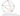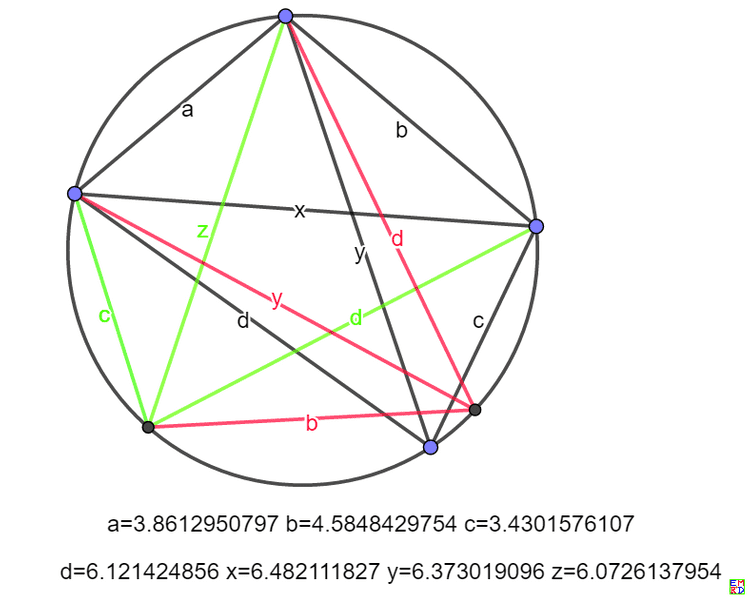## 圆内接四边形的三条对角线

Fri, 17th January 2020Edit on Githubdiagonal

# 问题溯源

wayne的微信群提出了一个恒等式问题 :
$a,b,c,d,x,y$为正实数，且满足$xy=ac+bd, \frac xy=\frac{ad+bc}{ab+cd}$

# 几何意义

mathe提议引入参数z并且设 $xz=ad+bc, yz=ab+cd$, “于是考虑边长为$a,b,c,d$的圆内接四边形，边顺序的不同安排得出对角线长度正好分别为$x,y;y,z;z,x$. 这时右边等式两边是不是有什么特殊的几何意义呢？” 通过利用托勒密定理：圆内接四边形对边乘积之和等于对角线乘积之和， 我们可以将恒等式中变量几何化# 利用对称性证明等式

hujunhua按照前面的思路，进一步把原题改造为对称形式

$\frac{abc+bcd+cda+dab+xyz}{a+b+c+d+x+y+z}$

# 派生的几何结论

dingjifen发现，如果圆内接四边形面积为S,那么$xyz=4RS$.

# 问题推广

wayne另外发现 a,b,c,d可以拓展到任意实数范围，只要分母非零，等式还是依旧成立，这时就不能用几何模型解释了。我们可以从解析函数的解析延拓角度去理解, 所以只要分母非零，等式对复数也会成立。

Github